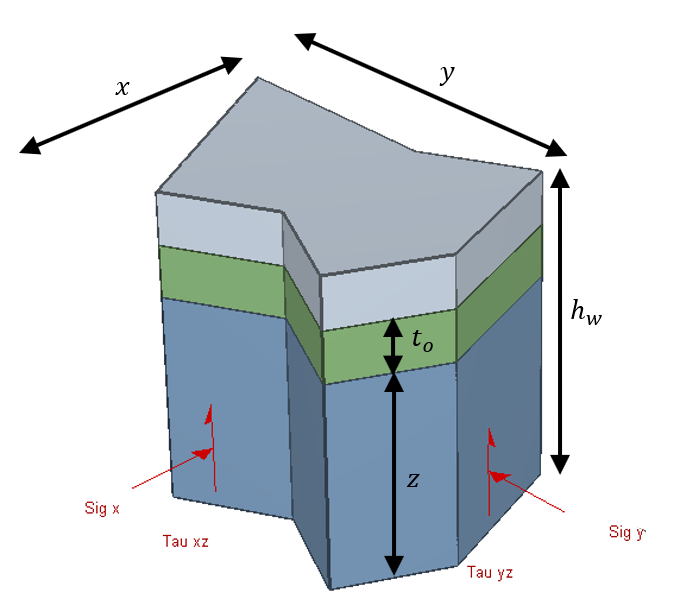The Rocscience International Conference 2021 Proceedings are now available. Read Now

Input Data Overview

CPillar computes the factor of safety for a rectangular crown pillar formed by:

• Some length (x) and width (y),
• Some uniform height (z), and
• Vertical abutments (for a rectangular pillar).

A typical rectangular problem geometry is illustrated below

Typical rectangular pillar geometry for CPillar analysis.CPillar computes the factor of safety for a polygonal crown pillar formed by:

• At least three unique vertices (x, y) that define a 2D polygon,
• Some uniform height (z), and
• Vertical abutments (for a rectangular pillar).

A typical polygonal problem geometry is illustrated below

Typical polygonal pillar geometry for CPillar analysis.After entering model inputs, CPillar checks if the geometry of a model is valid, before proceeding to calculate a Safety Factor or Probability of Failure for pillar stability. For more information on the validation of model geometry see the Geometry Overview topic.

To enter model parameters, select the Pillar Information section in the Sidebar. This section allows you to define the following model input parameters: